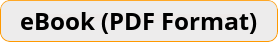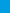FSA Mathematics Workbook For Grade 7 Step-By-Step Guide to Preparing for the FSA Math Test 2019FSA Mathematics Workbook is full of specific and detailed material that will be key to succeeding on the FSA Math. It's filled with the critical math concepts a student will need in order to ace the test. Math concepts in this book break down the topics, so the material can be quickly grasped. Examples are worked step–by–step, so you learn exactly what to do.\$12.99\$5.99
-54% only \$5.99

The Best Book to ACE the FSA Math Test!

The goal of this book is simple. It will help your student incorporates the best method and the right strategies to prepare for the FSA Mathematics test FAST and EFFECTIVELY.

FSA Mathematics Workbook is full of specific and detailed material that will be key to succeeding on the FSA Math. It’s filled with the critical math concepts a student will need in order to ace the test. Math concepts in this book break down the topics, so the material can be quickly grasped. Examples are worked step–by–step, so you learn exactly what to do.

FSA Mathematics Workbook helps your student to focus on all Math topics that students will need to ace the FSA Math test. This book with 2 complete FSA tests is all your student will ever need to fully prepare for the FSA Math.

This workbook includes practice test questions. It contains easy–to–read essential summaries that highlight the key areas of the FSA Math test. Effortless Math test study guide reviews the most important components of the FSA Math test. Anyone planning to take the FSA Math test should take advantage of the review material and practice test questions contained in this study guide.

Inside the pages of this workbook, students can learn basic math operations in a structured manner with a complete study program to help them understand essential math skills. It also has many exciting features, including:

• Dynamic design and easy–to–follow activities
• A fun, interactive and concrete learning process
• Targeted, skill–building practices
• Math topics are grouped by category, so students can focus on the topics they struggle on
• All solutions for the exercises are included, so you will always find the answers
• 2 Complete FSA Math Practice Tests that reflect the format and question types on FSA

FSA Mathematics Workbook is a breakthrough in Math learning — offering a winning formula and the most powerful methods for learning basic Math topics confidently. Each section offers step–by–step instruction and helpful hints, with a few topics being tackled each chapter. Two complete REAL FSA Math tests are provided at the back of the book to refine your student’s Math skills.

FSA Mathematics Workbook is the only book your student will ever need to master Basic Math topics! It can be used as a self–study course – you do not need to work with a Math tutor. (It can also be used with a Math tutor).

Ideal for self–study as well as for classroom usage.